16. Only one of the following statements is true. Which one?

17. The diagram is a “map” of Jo’s local rail network, where the dots represent stations and the lines are routes.

Jo wants to visit all the stations, travelling only by train, starting at any station and ending at any station, with no restrictions on which routes are taken.

What is the smallest number of stations that Jo must go to more than once?

A:
B:
C:
D:
E:

18. Which of these statements is true?

A: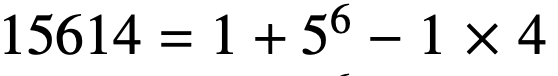B: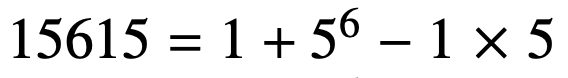C: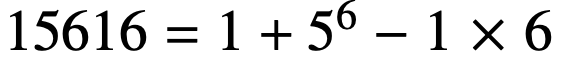D: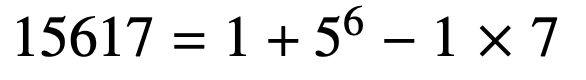E: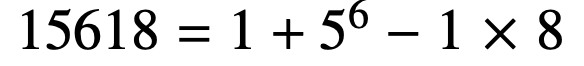19. Jack and Jill played a game for two people. In each game, the winner was awarded 2 points and the loser 1 point. No games were drawn.

Jack won exactly 4 games and Jill had a final score of 10 points. How many games did they play?

A:
B:
C:
D:

20. Box P has p chocolates and box Q has q chocolates, where p and q are both odd and p > q.

What is the smallest number of chocolates which would have to be moved from box P to box Q so that box Q has more chocolates than box P?

A: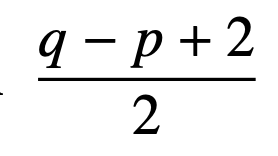B: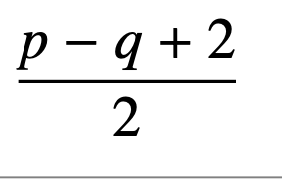C: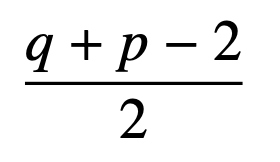D: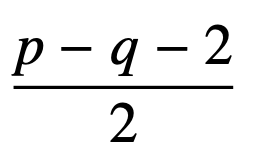E: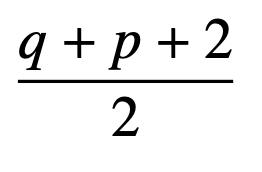21. Pablo’s teacher has given him 27 identical white cubes.

She asks him to paint some of the faces of these cubes grey and then stack the cubes so that they appear as shown.

What is the largest possible number of the individual white cubes which Pablo can leave with no faces painted grey?

A:B:C:D:E:22. In the division calculation 952 473 ÷ 18, which two adjacent digits should be swapped in order to increase the result by 100?

A:B:C:D:E:23. Sam wants to complete the diagram so that each of the nine circles contains one of the digits from 1 to 9 inclusive and each contains a different digit.

Also, the digits in each of the three lines of four circles must have the same total. What is this total?

A:B:C:D:E:24. The diagram shows a regular octagon with sides of length 1. The octagon is divided into regions by four diagonals.

What is the difference between the area of the hatched region and the area of the region shaded grey?

A: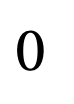B: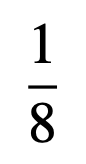C: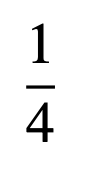D: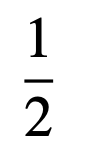E: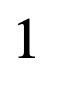25. A die has the shape of a regular tetrahedron, with the four faces having 1, 2, 3 and 4 pips.

The die is placed with 4 pips ‘face down’ in one corner of the triangular grid shown, so that the face with 4 pips precisely covers the triangle marked with 4 pips.

The die is now ‘rolled’, by rotating about an edge without slipping, so that 1 pip is face down. It is rolled again, so that 2 pips are face down, as indicated.

The rolling continues until the die rests on the shaded triangle in the opposite corner of the grid.

How many pips are now face down?

A:B:C:D:E:Back to the top